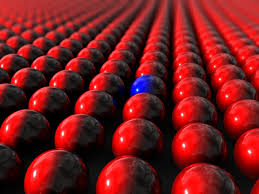# 10 BoxesThere are 10 boxes containing blue and red balls.

The number of blue balls in the $n^\text{th}$ box is given by $B(n) = 2^n$.
The number of red balls in the $n^\text{th}$ box is given by $R(n) = 1024 - B(n)$.

A box is picked at random, and a ball is chosen randomly from that box. If the ball is blue, and the probability that the $10^\text{th}$ box was picked can be expressed as $\frac ab$, where $a$ and $b$ are coprime positive integers, find $a+b$.

More probability questions

Photo credit: http://www.spi-global.com/

×